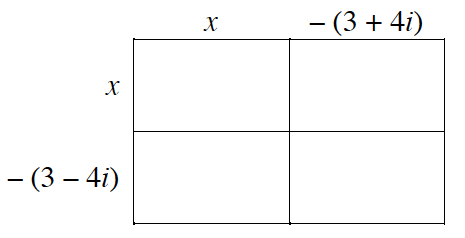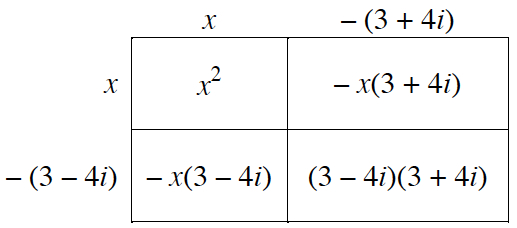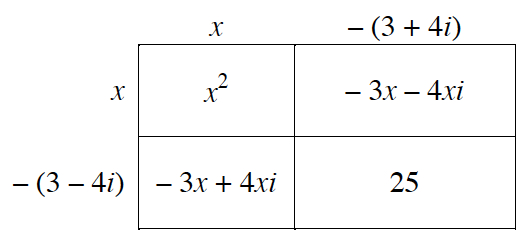### Home > PC3 > Chapter 4 > Lesson 4.1.3 > Problem4-43

4-43.

Phyllis has a problem. She knows that the roots of a quadratic function are $x=3+4i$ and $x=3-4i$, but in order to get credit for the problem she needs to know the equation of the function. She is having a hard time with the complex roots, but luckily, her friends are full of advice.

1. Mercedes says, “Just remember when we made factors from the roots. If the roots are $7$ and $4$ the equation is $y=(x-7)(x-4)$.” Use Mercedes’s idea to help Phyllis write the equation of a quadratic function.

$y=(x−(3+4i))(x−(3−4i))$

Generic rectangle, 2 rows, 2 columns, top edge labeled, x minus quantity, 3 + 4 I, left edge labeled, x, minus quantity, 3 minus 4 i.

Labels added: Interior, top left, x squared, to right, negative x times the quantity, 3 + 4 I, bottom left, negative x times the quantity, 3 minus 4 I, bottom right, quantity, 3 minus 4 I, times the quantity, 3 + 4 I.

Interior labels changed: top left, negative 3 x, minus 4 x I, bottom left, negative 3 x, + 4 x I, bottom right, 25.2. Darin says, “No, no, no. You can do it that way, but that’s too complicated. I think you just start with $x=3+4i$ and work backwards. So $x-3=4i$, then, square both sides… Yeah, that’ll work.” Try Darin’s method.

$(x−3)^2=(4i)^2$

3. Whose method do you think Phyllis should use? Explain your choice.